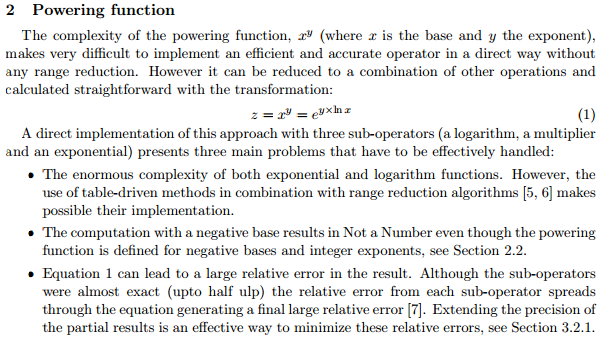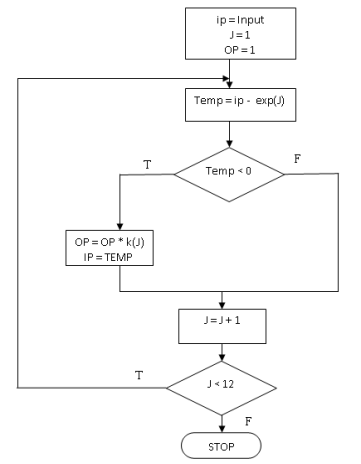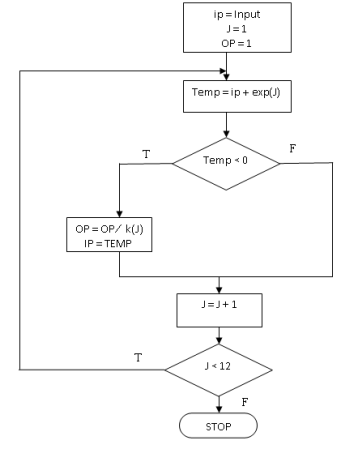# Modification of Power Series Expansion

1625 words (7 pages) Essay in Computer Science

18/07/18 Computer Science Reference this

Disclaimer: This work has been submitted by a student. This is not an example of the work produced by our Essay Writing Service. You can view samples of our professional work here.

Any opinions, findings, conclusions or recommendations expressed in this material are those of the authors and do not necessarily reflect the views of UK Essays.

The available methods to compute the logarithm of a number using digital circuits can be divided in two main groups. On the one hand, we have the look-up table based algorithms and, on the other, iterative methods. The first approach is faster and straightforward, but only useful for low precision. For implementing it, requires large amount of memory for increasing the accuracy. This is due to the size of the look-up table. We only evaluated iterative algorithms that need small look-up tables. The second group is slower, but suitable for high precision. Taylor’s series expansion is among the most popular methods to manually compute logarithms, but it has a slow convergence and requires slow operations like the division. Hence, they are slow when no embedded multipliers are available. Many studies explore hybrid implementations that take advantages from both groups.

Our project required an algorithm that could be implemented on FPGAs from any vendor. It should be platform independent. Our algorithm requires less memory and no multiplier at all to implement exponential and logarithm function.To the best of our knowledge there are only two previous works focused on the exponential function  , and only one for the logarithm function  (from the same authors of ).

The first one , employs an algorithm that does not exploit the FPGA characteristics, and consequently presents poor performance. The other two implementations [9, 10] are part of a common work and are designed suiting with FPGA flexibility (using internal tailored fixed arithmetic and exploiting the parallelism features of the FPGA) achieving much better results.

They are parameterizable implementations that, additionally to single f.p. format, also allow smaller exponent and mantissa bit-widths and are both based on input range reduction and table-driven methods to calculate the function in the reduced range. Our ex and ln x units, based on these units, include the following innovative features:

Single precision f.p. arithmetic extension. [9, 10] were designed considering only normalized numbers, not denormalized. Additional logic has been introduced to handle denormalized numbers at the output of ex and the input of ln x.

² Redesign of units to deal only with single precision. The feature of bit-width config-urability of the base designs has been removed. Thus, the resources needed have been reduced because specific units, just for single precision, have been developed.

² Simplification of constant multiplications. As suggested in , conventional multipliers have been removed where the multiplications involved constant coe±cients, improving performance and reducing size.

² Unsigned arithmetic. In [9, 10] internal fixed arithmetic with sign is used. However, some operations (like the ones involving range reduction and calculation of the exponent for the result in ex) are consecutive and related, and the sign of the result can be inferred from the input sign. For such operations signed arithmetic has been replaced by unsigned arithmetic with the corresponding logic reduction.

² Improved pipelining. The speed is enhanced by systematically introducing pipeline stages to the datapath of the exponential and logarithm units and their subunits

The paper  explains about the implementation of power and log function based on a simple modification of power series expansion of Taylor series. In power function implementation, the paper aims at reducing the exponent number to a smaller value. It requires a large amount of block ram and hardware multipliers as well. It becomes platform dependent and the clock frequency may vary from vendor to vendor. The degradation in throughput rate is due to the use of 18 X 18 embedded multipliers in it. The powering unit also requires more number of stages which may be reduced further.

In the proposed method, we are going to reduce delay and improve the throughput rate by avoiding the embedded multipliers and block RAMs. In this paper, we are not completely avoid look up tables, but any value of logarithm or exponential can be calculated, by adjusting the look up table values to the desired number

 C. C. Doss and R. L. Riley, FPGA-Based implementation of a robust IEEE-754 ex-ponential unit,” in IEEE Field-Programmable Custom Computing Machines, 2004, pp. 229{238.

 J. Detrey and F. de Dinechin, A parameterized °oating-point exponential function for FPGAs,” in IEEE International Conference Field-Programmable Technology, 2005, pp.27{34.

 ||, A parameterized °oating-point logarithm operator for FPGAs,” in Signals, Systems and Computers, 2005. Conference Record of the Thirty-Ninth Asilomar Conference, 2005, pp. 1186{1190.

 Pedro Echeverra, Marisa Lopez-Vallejo,”An FPGA Implementation of the Powering Function with Single Precision Floating-Point Arithmetic

“An FPGA Implementation of the Powering Function with Single Precision Floating-Point Arithmetic”

PROPOSED METHOD

The proposed method avoids multiplication and division operations, and is thus suitable for implementation in software on processors that lack such instructions (or where the instructions are slow) or in hardware on a programmable logic device or dedicated chip. This method is suitable when shifters are available in abundant. It is an extension to the implementation of sine and cosine explained in CORDIC. The proposed algorithm evaluates the power functions for both positive and negative values. There are some constants by which it is easy to multiply. For example, multiplying by 2n, where n is a positive or a negative integer, can be achieved by simply shifting a number by n places. The shift will be to the left (division) if n is positive, to the right (multiplication) if n is negative. It is nearly as easy to multiply by numbers of the form ±2n±1. These simply involve an add (or) subtract a shift.

Implementation of EXP:

For implementing y = exp(x). The algorithm is going to generate a sequence of values for x and y, and we are going to make sure that for each pair

 K Exp(k) 5.5452 256 2.7726 16 1.3863 4 0.6931 2 0.4055 3/2 0.2231 5/4 0.1178 9/8 0.0606 17/16 0.0308 33/32 0.0155 65/64 0.0078 129/128

y=exp(4)·

y′=exp(4)·exp(-(xk))

=exp(4)·exp(-x)·exp(k)

=y·exp(k).

In other words, if we subtractkfromx, we have to multiplyyby exp(k). All we have to do now is make sure that exp(k) is a nice number, so we can multiply by it easily, and the rest is straightforward. Note thatkitself does not have to be nice, as we are only subtracting that, not multiplying by it. Here are some nice values of exp(k) and the corresponding (not necessarily nice) values ofk.

The flow of algorithm is as follows for positive powers of x:Here in each iteration, we subtract the input from the nearest value of exp(k) as listed in the table. If the difference is negative, we multiply the output by the corresponding exp(k). The process continues withmore entities in our table of k, finally we get the result. In the same way the flow chart is mentioned for nagative powers of x.

 K Exp(k) 5.5452 256 2.7726 16 1.3863 4 0.6931 2 0.2877 3/4 0.1335 7/8 0.0645 15/16 0.0317 31/32 0.0157 63/64 0.0078 127/128 0.0039 255/256

The flow of algorithm is as follows for negative powers of x:Here in each iteration, we subtract the input from the nearest value of exp(k) as listed in the table. If the difference is positive, we divide the output by the corresponding exp(k). The process continues withmore entities in our table of k, finally we get the result.

Implementation of LOG :

For implementing Y=log (x), the procedure is similar to the implementation of exponential function

#### Cite This Work

To export a reference to this article please select a referencing stye below:

## Related Services

View all

### DMCA / Removal Request

If you are the original writer of this essay and no longer wish to have the essay published on the UK Essays website then please:Prices from
###### £28

Undergraduate 2:2 • 250 words • 7 day delivery

Delivered on-time or your money back

##### Rated 4.6 out of 5 by(146 Reviews)